# PN Junction diode:

### What is PN Junction diode?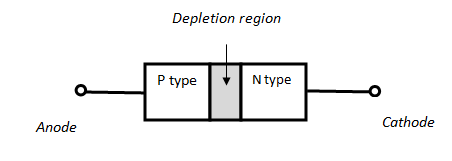PN Junction diode

PN Junction Diode is the most commonly used semiconductor device. As the name says PN Junction Diode is the interface of P type semiconductor material and N type semiconductor material.  P type is doped with trivalent impurity and the majority carrier is holes and N type is doped with pentavalent impurity and the majority carrier is electrons.

Very useful property of PN Junction diode is, it allows electrons to flow in only one direction and thus current flows in only one direction , in the other direction it does not allow current to flow. It is made up of semiconductor materials like Silicon, Germanium and Gallium Arsenide.

#### Symbol and polarity of PN Junction Diode:

As the name says  diode means “di”  is two and “odes” is short form of electrodes. So the two electrodes are cathode and anode. Anode is a positive terminal , represented as A and Cathode is a negative terminal represented as K.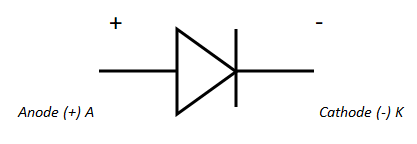Symbol and polarity of PN Junction diode

#### What is Depletion Region?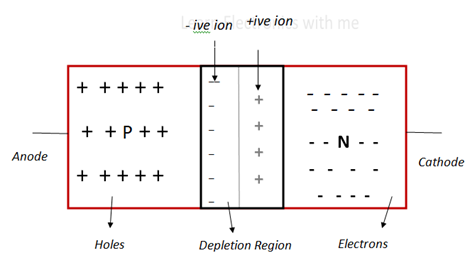Depletion region

When p type and n type semiconductor are interfaced ,the holes and the electrons flow from higher concentration area into lower concentration area. This is because of attraction towards the opposite charges.

The majority carrier in the N type is electrons which diffuses into the P type region and holes which is the majority carrier in the P type material diffuses into N type material . This forms the depletion region in the junction. Depletion means decrease in quantity of something.

#### Working of PN Junction diode:

Some of the holes from P region move to N region and some of the electrons from N region is move to P region. Ions are formed by losing or gaining electrons from the other atoms.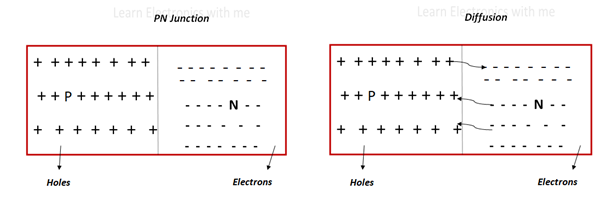So negative ions are formed in the P type region near the junction and positive ions are formed in the N type region near the junction. Negative ions have more number of electrons than protons and hence it is negatively charged.

Positive ions have more number of protons than electrons and hence positively charged. These charges formed in the junction are immobile. This forms like the barrier at the junction and does not allow free electrons or holes from moving to the other side.

#### Forward bias: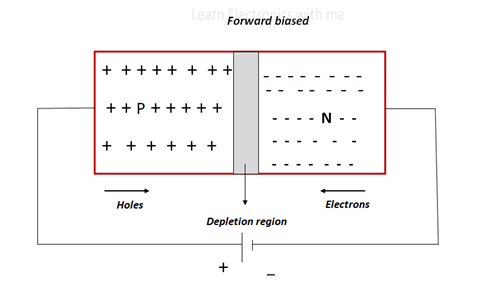Forward bias

Bias refers to the voltage or current applied to the terminals. In the forward bias the positive terminal is connected to the P type and negative terminal to the N type material. The value of the potential barrier of the depletion region for Si is 0.7v and for Ge is 0.3v.

When the applied external voltage is more than the potential barrier value, the free electrons and holes gets the extra energy to cross the depletion region and thus the depletion region decreases in size and the current starts to flow.

#### Reverse Bias: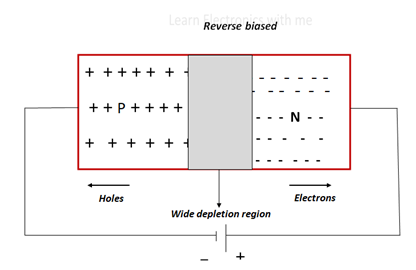Reverse bias

In the reverse bias negative terminal is connected to the P type and positive terminal to the N type. So the negatively charged electrons are attracted towards P type and positively charged holes moves to the N type and thus the size of the depletion increases. Thus the potential barrier across the junction does not allow the current to flow.

#### V-I  characteristics of PN Junction diode: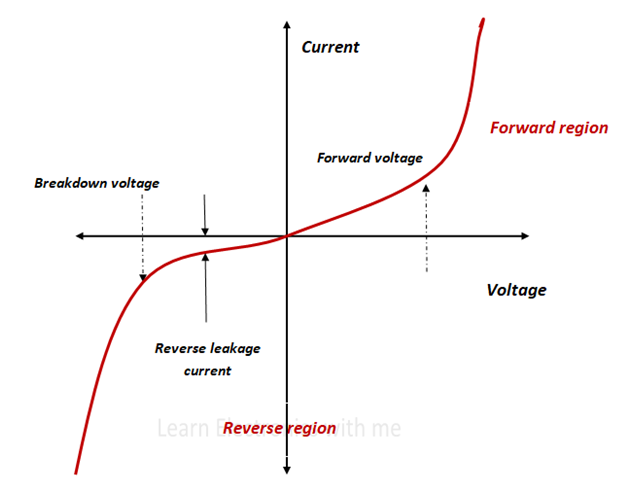V I characteristics of PN Junction diode

Current taken in the Y axis is the circuit current and the voltage taken in the X axis is the external voltage applied.

When the diode is forward biased, when the external applied voltage is increased and when it  crosses the value of potential barrier the current increases steadily as we see in the figure.

When the diode is reverse biased, only small current flows. As the applied voltage increases at a point it causes breakdown of the junction which is a permanent breakdown resulting in the sudden increase in the current.

• It is smaller in size and light weight
• It is very cheap
• It consumes less power and operates with low voltage source
• It operates very fast
• It has long life